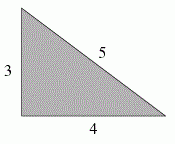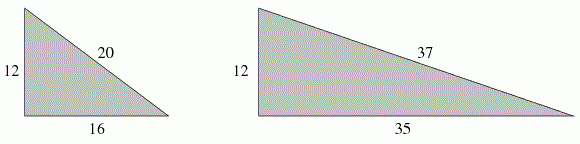favorite We need a little bit of your help to keep things running, click on this banner to learn more
Problems

# Pythagoras's Revenge

The famous Pythagorean theorem states that a right triangle, having side lengths A and B and hypotenuse length C, satisfies the formula A2 + B2 = C2. It is also well known that there exist some right triangles in which all three side lengths are integral, such as the classic:Further examples, both having A = 12, are the following:The question is, given a fixed integer value for A, how many distinct integers B > A exist such that the hypotenuse length C is integral?

#### Input

Each line contains a single integer A (2A < 1048576 = 220). The end of the input is designated by a line containing the value 0.

#### Output

For each value of A, output the number of integers B > A such that a right triangle having side lengths A and B has a hypotenuse with integral length.

Time limit 1 second
Memory limit 128 MiB
Input example #1
3
12
2
1048574
1048575
0

Output example #1
1
2
0
1
175

Source 2012 ACM North America, Mid-Central, Problem A